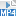## Content

### Stationary points

The stationary points of a graph $$y=f(x)$$ are those points $$(x,y)$$ on the graph where $$f'(x) = 0$$. A stationary point can be a turning point or a stationary point of inflexion.

Differentiating the term $$a_k x^k$$ in a polynomial gives $$k a_k x^{k-1}$$. So if a polynomial $$f(x)$$ has degree $$n$$, then its derivative $$f'(x)$$ has degree $$n-1$$. To find stationary points of $$y=f(x)$$, we must solve the polynomial equation $$f'(x)=0$$ of degree $$n-1$$.

Take an example from our gallery.

#### Example

Let $$f(x) = 2x^3 - 3x^2 + 5$$. Find the stationary points of the graph $$y=f(x)$$.

#### Solution

We compute $$f'(x) = 6x^2 - 6x$$. To find stationary points we solve $$6x^2 - 6x$$ = 0. Factorising to $$6x(x-1)=0$$ gives $$x=0$$ or $$x = 1$$. Substituting these values of $$x$$ gives $$f(0) = 5$$ and $$f(1) = 4$$. So the stationary points are $$(0,5)$$ and $$(1,4)$$.

Note. Here $$f$$ has degree 3, its derivative $$f'$$ has degree 2, and so $$f'(x)=0$$ is a quadratic equation.

The following exercise shows that a polynomial graph may have no stationary points. In this exercise, the polynomial $$f$$ again has degree 3 and its derivative $$f'$$ has degree 2, but the equation $$f'(x) = 0$$ has no solutions.

##### Exercise 8

Let $$f(x) = x^3 + x - 2$$. Show that $$f(x)$$ has no stationary points.

Screencast of exercise 8Next let's consider the number of stationary points of a polynomial graph $$y=f(x)$$, where $$f(x)$$ has degree $$n$$.

The stationary points are found by solving the equation $$f'(x)=0$$, which has degree $${n-1}$$, and hence has at most $$n-1$$ real solutions. Therefore the graph $$y=f(x)$$ has at most $$n-1$$ stationary points. This confirms our Conjecture 3.

Slightly more trickily, if the degree $$n$$ is even, then the degree $$n - 1$$ of the derivative $$f'(x)$$ is odd. So the graph of $$f'(x)$$ goes from $$-\infty$$ to $$+\infty$$, or vice versa. Therefore the graph of $$f'(x)$$ crosses the $$x$$-axis somewhere, changing sign from positive to negative or from negative to positive. This gives a turning point of $$f(x)$$. We have now confirmed Conjecture 4: when $$n$$ is even, the graph of $$f(x)$$ has at least one turning point.

The next exercise gives a test for the number of stationary points of a cubic polynomial.

##### Exercise 9
Let $$f(x) = ax^3 + bx^2 + cx + d$$, where $$a,b,c,d$$ are real numbers with $$a \ne 0$$. Show that:
1. If $$b^2 - 3ac < 0$$, then $$y=f(x)$$ has no stationary points.
2. If $$b^2 - 3ac = 0$$, then $$y=f(x)$$ has one stationary point.
3. If $$b^2 - 3ac > 0$$, then $$y=f(x)$$ has two distinct stationary points.

Next page - Content - Sketching polynomial functions